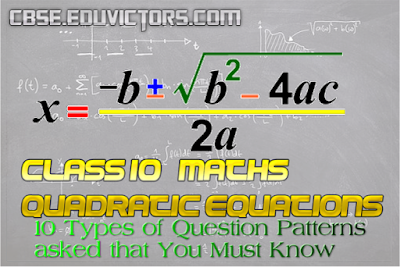## Quadratic Equations - 10 Types of Question Patterns asked that You Must Know

Here is a list of ten pattern types questions that are commonly asked in class 10 maths examination paper.  Solutions are also  provided in the end.

Q1: Determine whether the given values are solutions of the given equation or not?
x² − 3x + 2 = 0, x = 2, x = −1

Q2: Find the value of k for which the given value is a solution of the given equation
x² − x(a + b) + k = 0, x = a

Q3: The product of two consecutive positive integers is 306. Form the quadratic equation to find the integers, if x denotes the smaller integer.

Q4: Solve the give quadratic equation by factorization: (x - 4)(x + 2) = 0

Q5: Find the roots of the following quadratic equation (if they exist) by the method of completing
the square.
x² - 4√2 x +  6 = 0

Q6: Write the discriminant of the quadratic equation: 2x² - 5x + 3 = 0

Q7: Determine the nature of roots for the given quadratic equation:
x² + x + 2 = 0

Q8: Find the values of k for which the roots are real and equal for the given equation:
kx² + 4x + 1 = 0

Q9: (Word Problem) Find the consecutive numbers whose squares have the sum 85.

Q10: (Word Problem) A two-digit number is such that the products of its digits is 8. When 18 is subtracted from the number, the digits interchange their places. Find the number?

Solutions:
LHS = x² − 3x + 2
At x = 2,
LHS = 2² - 3(2) + 2 = 4 - 6 + 2 = 6 - 6 = 0 = RHS

At x =-1,
LHS = (-1)² -3(-1) + 2 = 1 + 3 + 2 = 6 ≠ RHS

∴ x = 2 is the solution of the given quadratic equation.

Given that x = a is a root of the given equation
x² − x(a + b) + k = 0
⇒ x = a satisfies the equation
⇒ (a)² − a(a + b) + k = 0
⇒ a² - a² - ab + k = 0
⇒ -ab + k = 0

Given that the smallest integer of 2 consecutive integer is denoted by x
⇒ The two integer will be x and (x + 1) and product is 306
⇒ x(x + 1) = 306
⇒ x² + x - 306 = 0         (answer)

Given (x - 4)(x + 2) = 0
⇒ Either x - 4 = 0        or x + 2 = 0
⇒ x = 4        or x = -2
Thus, x = 4 and x = -2 are two roots of the equation (x - 4)(x + 2) = 0

x² - 4√2 x +  6 = 0
x² - 2 × x × 2√2 + (2√2)² - (2√2)² + 6 = 0
(x - 2√2)² - (2√2)² + 6 = 0
(x - 2√2)² = (2√2)² - 6
(x - 2√2)² = 8 - 6
(x - 2√2)² = 2
x - 2√2 = ±√2
x - 2√2 = √2 OR x - 2√2 = -√2
x = 2√2 + √2 OR x = 2√2 -√2
x = 3√2 OR x =  √2 (answer)

2x² - 5x + 3 = 0
Here a = 2, b = -5 and c = 3
D = b² - 4ac
∴ D = (-5)² - 4(2)(3)
= 25 - 24

x² + x + 2 = 0
Here a = 1, b = 1, c = 2
D = b² - 4ac
D = (1)² - 4(1)(2)
= 1 - 8
= -7
As D < 0, the equation has no real roots.

kx² + 4x + 1 = 0
Here a = k,  b = 4 and c = 1
It is given that, the equation has real and equal roots
D = b² - 4ac = 0
(4)² - 4(k)(1) = 0
16 - 4k = 0
4k = 16

Let the two consecutive natural numbers be ‘x’ and ‘x + 1’
⇒ Given that the sum of their squares is 85
ATQ,
x² + (x + 1)² = 85
x² + x² + 2x + 1 = 85
2x² + 2x - 85 + 1 = 0
2x² + 2x - 84 = 0
x² + x - 42 = 0
x² + 7x - 6x - 42 = 0 (By mid term splitting)
x(x + 7) -6(x + 7) = 0
(x + 7)(x - 6) = 0
x + 7 = 0     or    x - 6 = 0
x = -7      or x = 6
Case I: x= -7
Ist number = -7
2nd number = -7 + 1 = -6

Case II: x = 6
Ist number = 6
2nd number = 6 + 1 = 7
The consecutive numbers are 6,7 and −6, −7. (answer)

Let the two digits be x and x – 2
Given that the product of their digits is 8.
⇒ x(x – 2) = 8
⇒ x 2 − 2x − 8 = 0
⇒ x 2 − 4x + 2x − 8 = 0
⇒ x(x − 4) + 2(x − 4) = 0
⇒ (x − 4)(x + 2) = 0
⇒ x = 4 or x = −2
Considering the positive value x = 4, x – 2 = 2.
∴ The two digit number is 42.